# Methods and formulas for coefficients in Fit Regression Model

Select the method or formula of your choice.

## Coefficient (Coef)

The formula for the coefficient or slope in simple linear regression is:

The formula for the intercept (b0) is:

In matrix terms, the formula that calculates the vector of coefficients in multiple regression is:

b = (X'X)-1X'y

### Notation

TermDescription
yiith observed response valuemean response
xiith predictor value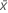mean predictor
Xdesign matrix
yresponse matrix

## Standard error of the coefficient (SE Coef)

For simple linear regression, the standard error of the coefficient is:

The standard errors of the coefficients for multiple regression are the square roots of the diagonal elements of this matrix:

### Notation

TermDescription
xiith predictor valuemean of the predictor
Xdesign matrix
X'transpose of the design matrix
s2mean square error

## T-value

### Notation

TermDescription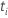test statistic for thecoefficient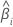estimated coefficient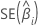standard error of theestimated coefficient

## P-value – Coefficients table

The two-sided p-value for the null hypothesis that a regression coefficient equals 0 is:

The degrees of freedom are the degrees of freedom for error, as follows:

np – 1

### Notation

TermDescription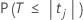The cumulative distribution function of the t distribution with degrees of freedom equal to the degrees of freedom for error.
tjThe t statistic for the jth coefficient.
nThe number of observations in the data set.
pThe sum of the degrees of freedom for the terms. The terms do not include the constant.
By using this site you agree to the use of cookies for analytics and personalized content.  Read our policy